### Force Class 8th Physics & Chemistry AP Board Solution

##### Question 1.What is a force? What changes can be produced by a force?Answer:Force is a physical (magnitude + direction) quantity which is represented as a ratio of change in momentum divided by change in time and is generally told as, force is a push or a pull that can change the state of the motion or can change the shape of the object on which force is applied. The strength of the force is called magnitude and there is always a direction mentioned along with the magnitude.The equation F= ma is the main equation used.The changes produced by the force are:-I. Force can stop a moving object.II. Force can initiate the motion of an object at rest.III. Force can change the shape of the object.IV. Force can change the direction of moving object.V. Force can change the speed of the object.VI. Force can rotate an object at rest or motion.Question 2.State an example of a situation mentioned below in which a force:a) changes the speed of an objectb) changes the shape of an objectc) changes the direction of an object.Answer:a) Kicking a football- we can make the football move from rest or we can change the speed of the moving ball by kicking it.b) Stretching a rubber band-when we stretch a rubber band its shape gets changed according to the applied force, we can enlarge it’s length or can make it small by squeezing it with some force.c) Cricket batsman-The batsman hits the ball with his choice he can either change the direction of moving ball entirely by hitting the ball towards baller or he can just touch the ball firmly or at the periphery to hit it sidewards.Question 3.How can you differentiate between a contact force and a force at a distance?Answer:To differentiate between these two forces we must first understand the meaning of them:-Contact forces- Those forces in which there is a physical contact between the surfaces on which force is applied.Noncontact forces-Those forces in which there is no physical contact between the surfaces and the force acts between them from a distance on which force is applied.Hence to differentiate between these two forces we must look for physical contact between the surfaces. If there is physical contact then it is a contact force otherwise it is non-contact force.Question 4.Give two examples each for a contact force and a force at a distance.Answer:Examples of contact and non-contact forces are :-a) CONTACT FORCE – Friction force(for this force to operate the two rough surfaces must be in contact) and Normal force(for this force to operate the contact of the object with the earth directly or indirectly must be there).b) NON-CONTACT FORCE – Magnetic force(we have seen magnets to repel and attract themselves without any contact between them) and Gravitational force(when we jump or when we throw something up earth exerts a force on us called gravity and pulls the thing down even when we are not in contact with earth).Question 5.Detect the errors in the following statement and rewrite it making necessary corrections.“Because the car is at rest, no forces are acting on it”.Answer:To get the statement correct we must understand the concept of net force . The resultant or the total sum of all the forces acting on the body are equal to the net force acting on that body , net force means total force acting on the body.Thus the statement can be corrected in two ways :-a) “Because the car is at rest, no net force is acting on it.” , orb) “Because the car is at rest, two forces are acting on it they are gravitational force(gravity) and the normal which sum up to zero.”Question 6.Why do tools meant for cutting always have sharp edges?Answer:Cuttingmeans the insertion of a thin object all along in a body so that the body gets divided into parts .Tools with thin or sharp edges allows us to concentrate or focus our applied force on less area through which the cutting of the object starts or we can say that the exerted pressure is more in case of thin tools.Whereas in case of thick tools the applied force gets exerted on a large area and hence the pressure is distributed over a large area so no penetration or insertion of the object happens, hence no cutting appears.Question 7.Objects change their state of motion due to the net force acting on them. Do you agree with this statement? Discuss with examples.Answer:If Fnet≠0, only then we can say that the state of motion can be changed, if Fnet=0 the state of motion can never change as force is the product of mass and acceleration and to change the speed of an object we must need acceleration. Yes, I agree with the statement.Question 8.When you push a heavy object, it doesn’t move. Explain the reason in terms of net Force.Answer:When any object is at rest and we try to move it the forces acting on the object are friction and the applied force by us. The object starts to move only when it’s Fnet≠0 and as we know that when the object is at rest and we apply force on it, the frictional force acting on it is the static friction which is not constant and is equal to the applied force till the object just starts to move and sliding friction takes over when the object moves properly.Question 9.If you push a heavy box which is at rest, you must exert some force to start its motion. However, once the box is sliding, you can apply a lesser force to maintain that motion. Why?Answer:When we try to push a heavy box which is at rest and as we know that when an object is at rest the frictional force acting on that is static friction which is not constant and static friction is equal to the applied force and increases as we increase the applied force till the object just starts to move and the maximum value of the static friction is greater than the value of sliding frictionwhich is constant and occurs when the object starts sliding or moving properly. The magnitude of sliding friction is always less than static friction.Question 10.Find net forces from the following diagrams.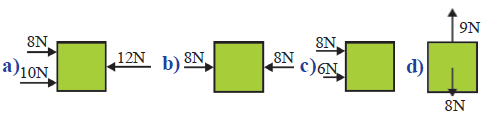Answer:As we need to calculate Fnet and we know that force is a quantity possessing magnitude and direction ,so, we need to consider the directions also with the numeric values of the forces applied on the blocks.The forces in opposite directions are to be subtracted from each other and the forces in same direction or parallel are to be added to each other. The resultant force will be in the higher magnitude force direction.a.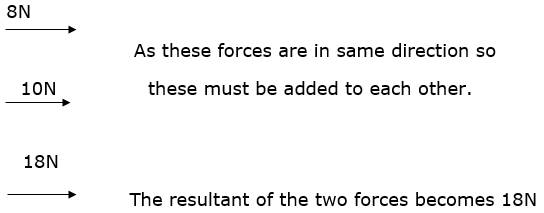b.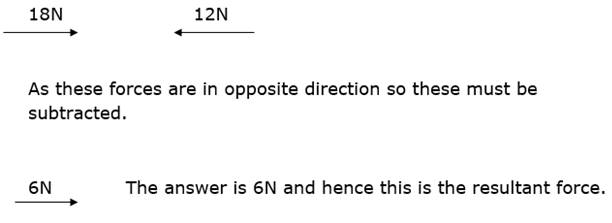c.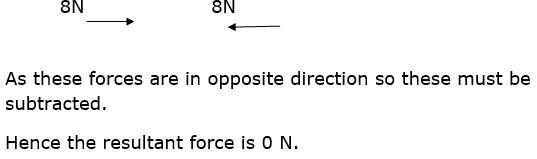d.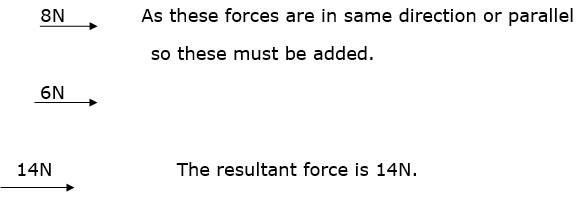e.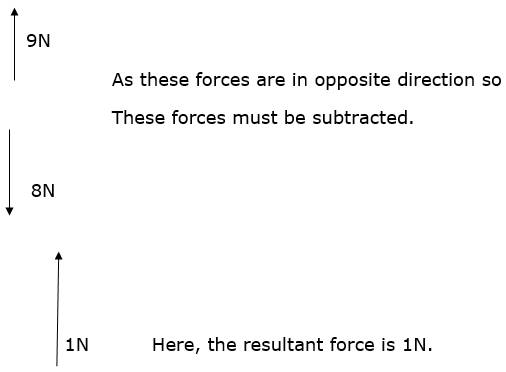Question 11.How do you increase the pressure by keeping (a) area unchanged and (b) force unchanged?Answer:Pressure is the ratio of force divided by area precisely the force counted is perpendicular to the area of consideration and not at any angle other than 90˚.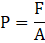As we know that to increase the value of fraction we must increase the value of numerator or decrease the value of denominator.a) To increase the pressure by keeping area constant or unchanged we must increase the force perpendicular to the area.b) To increase the pressure by keeping force constant or unchanged we must decrease the area of application.Question 12.Imagine that friction has disappeared from the earth. What will happen? Explain.Answer:When friction will disappear from the Earth, the following things will happen:-1. The brakes of the vehicles won’t work and the vehicles won’t run as the driving moving force is friction, the engine gives the rotating power to the wheels, the friction makes the car move forward.2. We won’t be able to walk because we walk when we apply force on the ground backward and the ground applies it’s friction force forward, hence we walk.3. We won’t be able to pick objects as the weight of the objects pulls it downward and the friction counter balances the weight and we are able to hold objects.4. We won’t be able to write with pencil. As we write on paper due to friction the pencil graphite gets traced on the paper etc.Question 13.Karthik is observing the live telecast of a one day cricket match. He noticed motion of a roller on the pitch during lunch break. He thought about various forces acting on the roller and the net force when it is in motion. Many questions arose in his mind regarding the direction of the net force. Can you guess what would be those questions?Answer: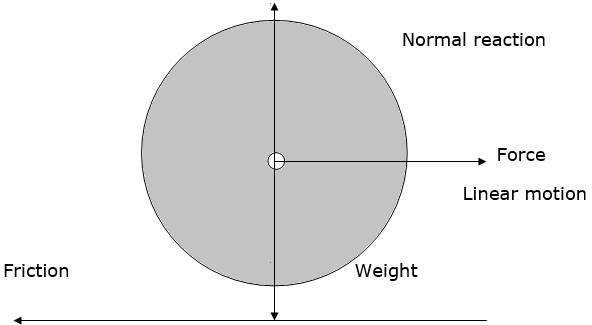i. What are the forces acting on roller when the roller is at rest and when it is moving?ii. Does the forces acting on the roller depend upon the direction of motion of the roller?iii. Can we get the direction of net force when force is applied on the roller by the people driving it?iv. Are there more than two net forces ?!Question 14.Design and conduct experiments to test few ways how friction may be reduced.Answer:Friction helps us to do various works but it is also evil , and to reduce this evil there are many ways that are:-a) Make the surfaces smoother by decreasing the roughness.b) Lubricating the surfaces.c) Make use of wheels that is use rolling friction rather than sliding friction.d) Reduce the contact and hence increase the space between the surfaces.e) Objects moving in fluids (liquids and gases) have streamline shapes to reduce friction by fluids called drag.f) In cars not only due to tyres we can reduce friction but due to the design of the car that is the streamlined car and the aerodynamics technology can reduce the air drag or the friction due to air(wind).EXPERIMENT-1,Smoother surfaces have less friction.Place a smooth inclined plane on a smooth table. Put a mark with a pen at any point A on the inclined plane. Now let a dry cell move down from this point. How far will it move , note the distance from the end of the inclined plane. Now place a cloth or sandpaper on the table , provided , they do not have any wrinkles on them. Now repeat the above steps i.e. make the dry cell move from the same point A and when it stops note the distance from the foot of the inclined plane till where it stops. You will observe that the cell moved larger distance in the case of smooth table surface and moved less distance in the cloth case and the least distance in the sand paper case because of friction. So we need smoother surfaces to reduce friction.EXPERIMENT-2,Rolling has less friction than sliding.Take a few pencils which are cylindrical(not hexagonal or triangular) in shape. Place them parallel to each other on a smooth table. Place a thick book over them and try to push the book , you will observe that the pencils roll when we push the book accordingly. Now try to push the book when it is kept on table directly , you will feel more effort is applied in this case than the pencil rolling one. So we need to use wheels to reduce friction.Question 15.a) Take two identical straws and suspend one of them freely. Rub the other straw with a piece of paper. Bring the rubbed end of the straw near the suspended one.What do you observe from this activity? Can you tell which type of force is it?b) Comb your dry hair. Bring the comb near small pieces of paper. What do you observe? Explain.Answer:There are forces of many types in the nature not only the muscular force of humans but also other forces between electric charges , magnets etc.a) When we do this activity we will observe that there is attraction between the two straws but not a strong one. This type of force is called electrostatic force. It is a non-contact force. It is formed due to induction of charge by friction or rubbing.But after some time this effect disappears.b) The pieces of paper gets attracted to the comb but the attraction is not very strong one. This type of force is called electrostatic force. It is due to when we rub the comb to our dry hair due to friction the comb gets induced charge and hence the charged comb attracts the bits of paper. But after some time the ability of the comb gets lost.Question 16.Collect pictures from various sources like internet, magazines, newspapers, etc, to illustrate contact forces, forces and prepare a scrap book.Answer:Examples of contact forces are :-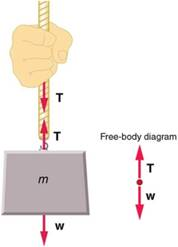Tension(pulling force)Frictional force or friction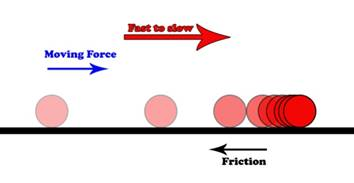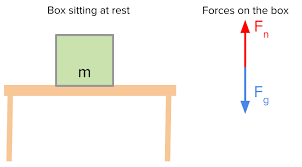Normal (reaction force) Fn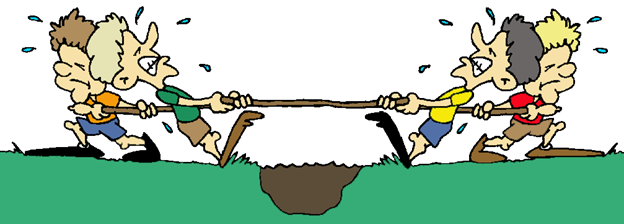Tug of war game, It has contact force mechanism.Question 17.A stick is placed on steps as shown in the figure. Draw normal forces on the stick.Answer: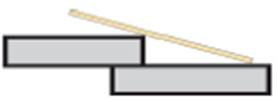As Normal is the reaction contact force which acts when there is some action force and Hence the Normal reaction force should be equal to the weight of the rod which is downward. In this situation the normal is not a operating on a single one but it is operating on 2 points in contact with the staircase.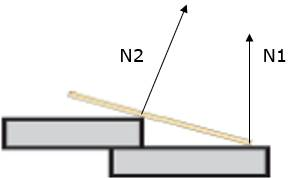N2 is slightly tilted towards N1 and N1 is straight and perpendicular to the staircase.Question 18.Observe the figure below and find in which direction the force of friction acts. Also indicate the normal forces and their directions.Answer: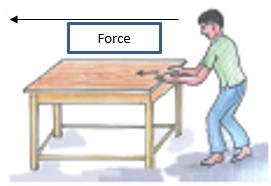The frictional force acts opposite to the direction of the applied force or the driving force applied by the boy on the table because frictional force prevents relative motion between two surfaces in contact.The normal reaction force is acting on every leg of the table and is equal in magnitude and the sum of the total normal reaction force is equal to the weight of the table acting at the centre of the table.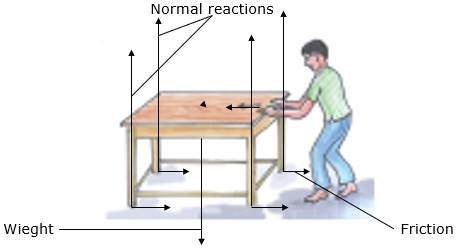The horizontal lines at the end of the legs of the table are the frictional forces.Question 19.A man is standing still on a level floor. What forces act on him? Draw a free bodydiagram (FBD) to show all forces acting on him.Answer: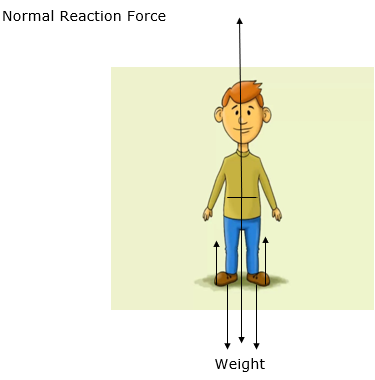If a man is standing still on the level floor the FBD of the man would have only two forces acting on the man , the forces are Weight of the man and the Normal reaction force of the man. The arrows pointing upwards are the normal force whereas the arrows pointing downwards are representing weight.If a man is walking the FBD of him would be completely different from this.Question 20.How do you appreciate the role of friction in facilitating our various activities?Answer:Frictional force or friction has many to do with our daily lives without the friction our life would be very difficult, but the activities friction facilitates are :-i. In walking friction through the ground give us a forward push as reaction when we push it.ii. In writing when we have to draw something beautiful, friction is there when we move our pencil on the paper the graphite of the pencil gets on the paper by friction and the pressure applied.iii. In vehicle motion and breaks the friction on the roads is the one that helps us to move our vehicle from one place to another and helps us to apply breaks whenever we want to , if we drive on icy roads where there is almost no friction the our car won’t run and also won’t stop properly.These are the general things we do in our daily lives in which friction is involved and plays very important role.Drifting of a car due to friction.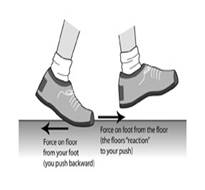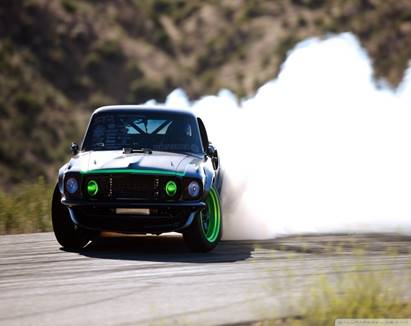Question 21.A monkey hangs stationary at the end of the vertical vine. What forces act on the monkey?Answer: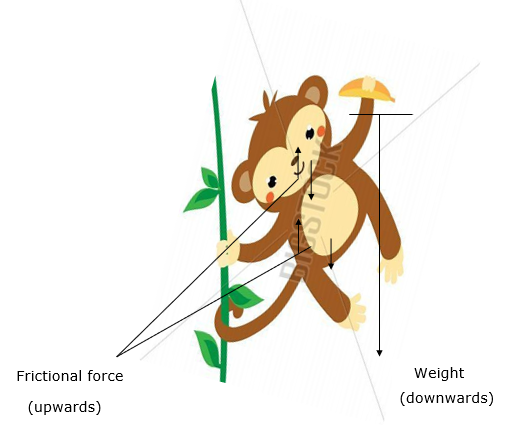Downward arrows are the weight and the upward arrows are frictionThe weight of the monkey and the frictional force from the contact(which depends on the strength with which monkey is holding the vein, more the strength more the friction ), the friction will increase with normal force of vertical vein and the monkey hand and tail , act on the monkey. Weight acts on the full body but is felt by the contact parts , in this case it is monkey’s hands.These are the two forces acting on the monkey.It is in the vertical case only and also if the weight of the monkey is on the tail the there is a pulling force in the tail of the monkey also.

PDF FILE TO YOUR EMAIL IMMEDIATELY PURCHASE NOTES & PAPER SOLUTION. @ Rs. 50/- each (GST extra)

HINDI ENTIRE PAPER SOLUTION

MARATHI PAPER SOLUTION

SSC MATHS I PAPER SOLUTION

SSC MATHS II PAPER SOLUTION

SSC SCIENCE I PAPER SOLUTION

SSC SCIENCE II PAPER SOLUTION

SSC ENGLISH PAPER SOLUTION

SSC & HSC ENGLISH WRITING SKILL

HSC ACCOUNTS NOTES

HSC OCM NOTES

HSC ECONOMICS NOTES

HSC SECRETARIAL PRACTICE NOTES

# 2019 Board Paper Solution

HSC ENGLISH SET A 2019 21st February, 2019

HSC ENGLISH SET B 2019 21st February, 2019

HSC ENGLISH SET C 2019 21st February, 2019

HSC ENGLISH SET D 2019 21st February, 2019

SECRETARIAL PRACTICE (S.P) 2019 25th February, 2019

HSC XII PHYSICS 2019 25th February, 2019

CHEMISTRY XII HSC SOLUTION 27th, February, 2019

OCM PAPER SOLUTION 2019 27th, February, 2019

HSC MATHS PAPER SOLUTION COMMERCE, 2nd March, 2019

HSC MATHS PAPER SOLUTION SCIENCE 2nd, March, 2019

SSC ENGLISH STD 10 5TH MARCH, 2019.

HSC XII ACCOUNTS 2019 6th March, 2019

HSC XII BIOLOGY 2019 6TH March, 2019

HSC XII ECONOMICS 9Th March 2019

SSC Maths I March 2019 Solution 10th Standard11th, March, 2019

SSC MATHS II MARCH 2019 SOLUTION 10TH STD.13th March, 2019

SSC SCIENCE I MARCH 2019 SOLUTION 10TH STD. 15th March, 2019.

SSC SCIENCE II MARCH 2019 SOLUTION 10TH STD. 18th March, 2019.

SSC SOCIAL SCIENCE I MARCH 2019 SOLUTION20th March, 2019

SSC SOCIAL SCIENCE II MARCH 2019 SOLUTION, 22nd March, 2019

XII CBSE - BOARD - MARCH - 2019 ENGLISH - QP + SOLUTIONS, 2nd March, 2019

# HSCMaharashtraBoardPapers2020

(Std 12th English Medium)

HSC ECONOMICS MARCH 2020

HSC OCM MARCH 2020

HSC ACCOUNTS MARCH 2020

HSC S.P. MARCH 2020

HSC ENGLISH MARCH 2020

HSC HINDI MARCH 2020

HSC MARATHI MARCH 2020

HSC MATHS MARCH 2020

# SSCMaharashtraBoardPapers2020

(Std 10th English Medium)

English MARCH 2020

HindI MARCH 2020

Hindi (Composite) MARCH 2020

Marathi MARCH 2020

Mathematics (Paper 1) MARCH 2020

Mathematics (Paper 2) MARCH 2020

Sanskrit MARCH 2020

Sanskrit (Composite) MARCH 2020

Science (Paper 1) MARCH 2020

Science (Paper 2)

Geography Model Set 1 2020-2021

MUST REMEMBER THINGS on the day of Exam

Are you prepared? for English Grammar in Board Exam.

Paper Presentation In Board Exam

How to Score Good Marks in SSC Board Exams

Tips To Score More Than 90% Marks In 12th Board Exam

How to write English exams?

How to prepare for board exam when less time is left

How to memorise what you learn for board exam

No. 1 Simple Hack, you can try out, in preparing for Board Exam

How to Study for CBSE Class 10 Board Exams Subject Wise Tips?

JEE Main 2020 Registration Process – Exam Pattern & Important Dates

NEET UG 2020 Registration Process Exam Pattern & Important Dates

How can One Prepare for two Competitive Exams at the same time?

8 Proven Tips to Handle Anxiety before Exams!